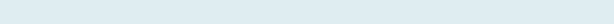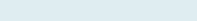# Average Top nth values

Suppose that you have a list of numbers and users want the average of top (Max)  numbers like first, fourth, sixth or any highest or lowest values from the array.

Query: We have Month and Employee Name with sales. Our task is to find the average of third, fourth, fifth and sixth largest sales in the given range.

Formula to implement:

=AVERAGE(LARGE(C3:F8,ROW(3:6)))

Functions applicable:

Average: Get an average of numbers positioned in one or multiple ranges.

Large: The Excel LARGE function returns the highest numeric value in the given range based on their position (k) i.e. the top value in the array.

Row: The Excel ROW function returns the row number of a reference cell

Formula Explanation (step by step)

Use shortcut F9 function key in Excel, tab clicks on evaluating the formula.

Let’s break the formula into multiple small steps to understand its application.

First formula

Syntax: =ROW([reference])

Step1: ROW(3:6)

Result: 3,4,5 and 6.

It will give row numbers 3, 4, 5 and 6.

Second formula

LARGE(C3:F8,ROW(3:6))

Syntax: LARGE(array,k)

Step 2:Result: 383,381,355, 350

As we already get the row number third, fourth, fifth, and sixth, now it returns highest number in the given range (C3:F8).

Third formula

=AVERAGE(LARGE(C3:F8,ROW(3:6)))

Syntax: AVERAGE(number1, [number2], …)

Step3:Result: 367.25

It will give the average number of selected top numbers based on the rank (k).

Note: In case you want the average of lowest nth numbers from the array to apply Small instead of Large in the formula.

For more knowledge and understanding please go through Average based on condition.